Get inspired by the success stories of our students in IIT JAM MS, ISI  MStat, CMI MSc DS.  Learn More

# ISI MStat 2019 PSA Problem 14 | Reflection of a pointThis is a problem from ISI MStat 2019 PSA Problem 14. First, try the problem yourself, then go through the sequential hints we provide.

## Reflection of a point - ISI MStat Year 2019 PSA Question 14

The reflection of the point (1,2) with respect to the line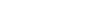is

• (3,6)
• (6,3)
• (10,5)
• (5,10)

### Key Concepts

Straight line

ISI MStat 2019 PSA Problem 14

Precollege Mathematics

## Try with Hints

Find an algorithm to find the reflection,

Find the line perpendicular tothrough (1,2).
Find the point of intersection.
Use Midpoint Segment Result.

The line perpendicular tois of the form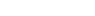.Now it passes through (1,2) . So,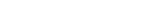Hence the line perpendicular tothrough (1,2) is y=2x.

Now we will find point of intersection (Foot of Perpendicular )

(3,6) is the point of intersection i.e the foot of perpendicular.

Use Mid-Point Formula (special case of Section formula) to get required point (Foot of perpendicular is mid-point of reflection and original point)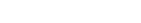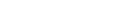Therefore the reflection point is (5,10) .

## Subscribe to Cheenta at Youtube

This is a problem from ISI MStat 2019 PSA Problem 14. First, try the problem yourself, then go through the sequential hints we provide.

## Reflection of a point - ISI MStat Year 2019 PSA Question 14

The reflection of the point (1,2) with respect to the lineis

• (3,6)
• (6,3)
• (10,5)
• (5,10)

### Key Concepts

Straight line

ISI MStat 2019 PSA Problem 14

Precollege Mathematics

## Try with Hints

Find an algorithm to find the reflection,

Find the line perpendicular tothrough (1,2).
Find the point of intersection.
Use Midpoint Segment Result.

The line perpendicular tois of the form.Now it passes through (1,2) . So,Hence the line perpendicular tothrough (1,2) is y=2x.

Now we will find point of intersection (Foot of Perpendicular )

(3,6) is the point of intersection i.e the foot of perpendicular.

Use Mid-Point Formula (special case of Section formula) to get required point (Foot of perpendicular is mid-point of reflection and original point)Therefore the reflection point is (5,10) .

## Subscribe to Cheenta at Youtube

This site uses Akismet to reduce spam. Learn how your comment data is processed.

### Knowledge Partner